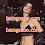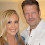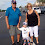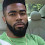## Friday 6 September 2013

### Correlations

In this post, two types of Correlations will be discussed, the non-parametric correlation called Spearman's Rank Correlation, and the parametric correlation called the Pearson Correlation.

Spearman's Rank Correlation

Spearman's Rank Correlation $\rho$ is a non-parametric measure of the relationship between two variables, and tests whether these variables have a monotonic relationship.

Suppose two populations, with the same number of samples, have a monotonic relationship. If these two populations were paired together, and the pairs sorted by the magnitude of the first element in ascending order, the second elements would be ordered by magnitude in ascending order too. Conversely if the first element of the pair was used to order the pairs in descending order, the second element of the pair would be ordered in descending order too. This relationship would hold true if we  ordered the second element of the pair, and examined the ordering of the first element.  Thus, each of the pair of samples would be ordered in the same direction.

If two populations are anti-monotonic with respect to each other, then if these two populations were paired together, and the pairs sorted by the magnitude of the first element in ascending order, the second elements would be ordered by magnitude in descending order. Conversely if the first element of the pair was used to order the pairs in descending order, the second element of the pair would be ordered in  ascending order. This relationship would hold true if we ordered the second element of the pair, and examined the ordering of the first element.  Thus, each of the pair of samples would be ordered in the opposite direction.

Spearman's Rank Correlation is bounded in value, between $-1$ and $+1$, for when the variables are related in either an anti-monotonic or monotonic manner with each other respectively, and is given by the following equation:-

$\Large \rho=\frac{\sum_{i=1}^{n}{(x_i-\bar{x})(y_i-\bar{y})}}{\sqrt{\sum_{i=1}^{n}(x_i-\bar{x})^2\sum_{i=1}^{n}(y_i-\bar{y})^2}}$ (Eq. 1)

where $x_i$ and $y_i$ are the result of converting $n$ (raw) samples $x[i]$ and $y[i]$ to their ranks respectively, and $\bar{x}$ and $\bar{y}$ are the mean of the ranks $x_i$ and $y_i$ respectively.

If there are no duplicate values of the raw samples ($x[i]$ and $y[i]$), i.e. no tied ranks, the above formula (Eq. 1) simplifies to

$\Large \rho=1-\frac{6\sum_{i=1}^{n}d_i^2}{n(n^2-1)}$ (Eq. 2)

where $d_i=x_i-y_i$. A derivation of this follows.

When there are no ties

$\sum_{i=1}^{n}(x_i-\bar{x})^2=\sum_{i=1}^{n}(y_i-\bar{y})^2$ (Eq. 3)

Also, $\bar{x}=\bar{y}$ regardless of whether there are ties or not.

Expanding the left hand side of (Eq. 3), we have

$\large \sum_{i=1}^{n}(x_i-\bar{x})^2=\sum_{i=1}^{n}(x_i^2-2x_i\bar{x}+\bar{x}^2)$

$\large =\frac{n(n+1)(n+2)}{6}-\frac{n(n+1)^2}{2}+\frac{n(n+1)^2}{4}$

$\large =\frac{n(n^2-1)}{12}$ (Eq. 4)

where we have used the identities

$\large \sum_{i=1}^{n}x_i^2=1^2+2^2+...+n^2=\frac{n(n+1)(n+2)}{6}$

and

$\large \sum_{i=1}^{n}x_i=1+2+...+n=\frac{n(n+1)}{2}$

Thus, the denominator of (Eq. 1) is $\large \frac{n(n^2-1)}{12}$.

Examining the numerator of (Eq. 1), we can expand this to

$\sum_{i=1}^{n}(x_i-\bar{x})^2$ - $\sum_{i=1}^{n}(x_i-\bar{x})^2$ + $\sum_{i=1}^{n}(x_i-\bar{x})(y_i-\bar{y})$

Using (Eq. 3) , we can further expand the numerator  to

$\sum_{i=1}^{n}(x_i-\bar{x})^2$ - $0.5\sum_{i=1}^{n}(x_i-\bar{x})^2$ $0.5\sum_{i=1}^{n}(y_i-\bar{y})^2$  + $\sum_{i=1}^{n}(x_i-\bar{x})(y_i-\bar{y})$

Dividing the expanded numerator by the denominator, (Eq. 1) becomes

$\Large \rho=1-\frac{\sum_{i=1}^{n}(x_i-\bar{x}-y_i+\bar{y})^2}{2\sum_{i=1}^{n}(x_i-\bar{x})^2}$

Substituting (Eq. 4), we have

$\Large \rho=1-\frac{6\sum_{i=1}^{n}(x_i-y_i)^2}{n(n^2-1)}$

If we denote $d_i=x_i-y_i$, we end up with

$\Large \rho=1-\frac{6\sum_{i=1}^{n}d_i^2}{n(n^2-1)}$

which is the simplified expression for $\rho$ when no ties are present.

As Spearman's Rank Correlation is a non-parametric test, it will be robust to the presence of outliers in the datasets.

Spearman's Rank Correlation - A worked example

Consider the raw sample pairs ($x[i]$,$y[i]$) as below

1 , 1
5 , 4
3 , 2
3 , 3

Their ranks ($x_i$,$y_i$) are on the right:-

($x[i]$,$y[i]$)    ($x_i$,$y_i$)      ($x_i$-$\bar{x}$)     ($y_i$-$\bar{y}$)
1 , 1         1, 1          -1.5         -1.5
5 , 4         4, 4           1.5           1.5
3 , 2      2.5, 2              0          -0.5
3 , 3      2.5, 3              0           0.5

The numerator of $\rho$ is ($-1.5 \times -1.5 + 1.5 \times 1.5 + 0 \times -0.5 + 0 \times 0.5$), which is $4.5$. The denominator of $\rho$ is $\sqrt{(2.25 + 2.25 + 0 + 0) \times (2.25 + 2.25 + 0.25 + 0.25)}$ which is $4.743416$.  Thus $\rho$ is $4.5/4.743416$, which is $0.948683$.

There is a Spearman Rank Correlation Calculator in this blog, which can be found here.

Pearson Correlation

The Pearson Correlation is the parametric equivalent of the Spearman Rank Correlation, and is given by the equation

$\Large r=\frac{\sum_{i=1}^{n}{(x[i]-\bar{x})(y[i]-\bar{y})}}{\sqrt{\sum_{i=1}^{n}(x[i]-\bar{x})^2\sum_{i=1}^{n}(y[i]-\bar{y})^2}}$

where $x[i]$ and $y[i]$ are the raw samples from the two populations that are to be correlated against, and $\bar{x}$ and $\bar{y}$ their respective means.

Like the Spearman Rank Correlation, the Pearson Correlation has a minimum of $-1$ and a maximum of +1. Unlike the Spearman Rank Correlation, it is sensitive to the value of the sample, and will be $+1$ only if the two populations are exactly linearly related with positive gradient, and $-1$ only if the two populations are exactly linearly related with negative gradient.

It is worth noting that the square of the Pearson Correlation ($r^2$) is the Coefficient of Determination, that was encountered in the Linear Regression post, in this blog. There is a Pearson Correlation Calculator in this blog, which can be found here

1.All thanks to Dr Raymond for helping me to get my penis longer and bigger with his cream in just two week his cream is very active and also it work fast for everyone who have used it, the best thing about his herbal cream is that it does not have side effects and the results is permanent. Note he can also cure the below sicknesses and disease PREMATURE EJACULATIONLOW SPERM COUNTERECTILE DYSFUNCTIONHIV/AIDS CUREHERPES CUREINFECTION CUREPILESTROKEHEART FAILUREYou can email him if you need his help via: drraymondherbalcenter@gmail.com or WhatsApp him via: +2348116744524

2.Especially superb!!! Exactly when I search for this.I found this webpage at the top of every single online diary in web crawler.

My web page ::: 휴게텔
(jk)

3.I'm 40 years old female I tested genital herpes (HSV1-2) positive in 2016. I was having bad outbreaks. EXTREMELY PAINFUL. I have try different kinds of drugs and treatment by the medical doctors all to know was avail. Six months ago I was desperately online searching for a helpful remedies for genital herpes (HSV1-2) cure, which I come across some helpful remedies on how Dr OYAGU have help so many people in curing genital herpes (HSV1-2) with the help of herbal treatment because I too believe there is someone somewhere in the world who can cure herpes completely. At of the past 2 months, however, I've been following his herpes protocol Via oyaguherbalhome@gmail.com or +2348101755322 and it stopped all outbreaks completely! To my greatest surprise I was cured completely by following the protocol of his herbal medicine . Don't be discouraged by the medical doctors. There is a cure for HSV with the help of herbs and roots by a herbalist Dr call Dr OYAGU he is so kind and truthful with his herbal treatment, kindly contact him for more information Via oyaguherbalhome@gmail.com or WhatsApp DR on +2348101755322 or visit his website https://oyaguspellcaster.wixsite.com/oyaguherbalhome you will be lucky as I am today

4.God is Good! I promised God that I would share my testimony on this blog. I had all the signs of STD Virus but I was not too sure as to which one. I did a lot of online research and scared myself straight for a whole week before going to see the nurse. She took one look at my genital part and first said that it could just be the anatomy of my body, then she said it looked like genital warts and that I may have herpes. I was devastated. She gave me some medicine for the herpes and some cream for the warts. I was also tested for every single STD including herpes. I went home and cried searching the web for all sorts of cures for herpes and awaiting my results. I saw a post whereby Dr. Oyagu cured Herpes and other diseases, I copied his contacts out and added him on whats app via (+2348101755322). The next day my test result was ready and i confirmed Herpes positive. I told Dr.Oyagu about my health problems and he assured me of cure. He prepared his herbal medicine and sent it to me. I took it for 14 days (2 weeks). Before the completion of the 14 days in which I completed the dose, the Blisters and Warts that were on my body was cleared. I went back for check-up and I was told I'm free from the virus. Dr. Oyagu cures all types of diseases and viruses with the help of his herbal medicine. You can reach Dr. Oyagu via his email address on (oyahuherbalhome@gmail.com) or WhatsApp him on (+2348101755322) Visit His website on https://oyaguspellcaster.wixsite.com/oyaguherbalhome

5.I'm so excited to share my testimonies about the Good Work of Dr odoma who cured my HIV/AIDS with his herbs, I never thought that I would live on earth before the year runs out. I have been suffering from a deadly disease (HIV) for the past (2)years now; I have spent a lot of money going from one Hospital to another looking for ways to get rid of this disease, the hospital has been my home everyday. Constant checks up have been my hobby not until this fateful day, I was searching
through the internet, I saw a testimony on how Dr Odoma helped someone in curing his HIV disease using his healing
Herbs, quickly I copied his email just to give him a test I spoke to him, he asked me to a preparation for the herbs items, of which I did exactly what he instructed me to do, he told me that he is going to provide the herbal cure to me, which he
did, few days after i went back to the same hospital that previously confirmed me as HIV/AIDS positive and this time the result declared me negative to the deadly disease of HIV/AIDS....so I decided to share my testimony, that nothing is impossible with God, God use a man to healed me. No matter what you are passing through, no matter how deadly the sickness is and no matter what the situation is, God that did mine is still going to do yours, suffering from AIDS, ALS, asthma.. Cancer, any kind of disease, you can reach him now via ? Gmail address: (DR odomasolutionhome20@gmail. com) WhatsApp number (+2348100649947)

6.I got diagnose of herpes virus last year, and i was taking some drugs prescribed for me by my family doc the drugs could not work and the herpes in my system was very terrifying that i was so depressed the good news is that i never gave up in searching for natural cure for herpes virus ,cause i believe so much in herbal medication.. One faithful morning i read a comment from a lady called Destiny Hudson on how she was cured with natural herbs made by DR.OYAGU from somewhere is West Africa, i immediately copied out his contact email via oyaguherbalhome@gmail.com and explain all my herpes problem to him via his email. the big truth is that it took just two week for his herbal medication to cure me completely without side affect. Till date am herpes virus free and all thanks to Dr OYAGU for his good deeds for me, Once again am very happy to share this great testimony of DR OYAGU cure hurry up now and contact him via his email address (oyaguherbalhome@gmail.com Call & WhatsApp him on (+2348101755322 ) and see what he can do

7.Life is always beautiful when you have good health.i have be in pain for almost 1 year had HSV 1&2 and I was lonely and sad, luckily I was directed to a very kind and Great Dr Oliha who helped me cure my HSV 1&2 and today I am free from the virus and very healthy thank you so much Dr Oliha .Email
oliha.miraclemedicine@gmail.com
you can also whatsapp/Call him; +2349038382931